Mathematics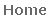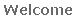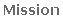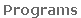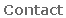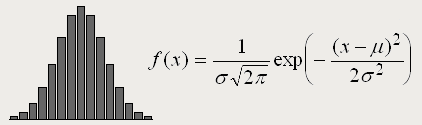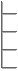"It is not knowledge, but the act of learning, not possession but
the act of getting there, which grants the greatest enjoyment.
When I have clarified and exhausted a subject, then I turn away
from it, in order to go into darkness again. The never-satisfied
man is so strange; if he has completed a structure, then it is not
in order to dwell in it peacefully, but in order to begin another. I
imagine the world conqueror must feel thus, who, after one
kingdom is scarcely conquered, stretches out his arms for
others."
 Carl Friedrich Gauss1777-1855
Our mathematics program offers rigorous training in analysis, logic, problem-solving and
technique. We offer programs in three streams:
 Level
 Course Outline
 Elementary MathematicsWhole Numbers, Decimals and Fractions, Integers, Algebra, Sequences,Ratios, Rates, Percents, Averages, Exponents, Plane Geometry
 Grade 9 Academic MathematicsAlgebra, Coordinate Systems, Polynomials, Exponent Laws, Slope andRate of Change, Equation of a Line, Linear Systems, Polygons and 3D Figures,Surface Area and Volume
 Grade 10 Academic MathematicsExpanding and Factoring, Linear Systems, Analytic Geometry, Equation for a Circle, Tangents, Quadratic Equations, Graphing Quadratics, Solving Systems of Equations, Trigonometry of Right Triangles, The Sine and Cosine Laws
 Grade 11 FunctionsRelations and Functions, The Inverse Function, Transformations, Polynomials,Rational Functions, Radicals, Linear-Quadratic Systems, Exponential Functions, Sinusoidal Functions, Sequences and Series, Discrete Functions, Financial Problems
 Grade 12 Advanced FunctionsFunctions, Systems of Equations, Polynomial Equations and Inequalities, Rational Functions, Absolute Value Functions, Trigonometric Functions and Identities, Exponential and Radical Functions, Logarithmic Functions, Composite Functions
 Grade 12 Calculus and VectorsLimits, Derivatives, Techniques and Applications of Derivatives, Curve Sketching,Differentiation of Exponential, Logarithmic and Sinusoidal Functions,Vectors, Equation of Lines, Equation of Planes, Integrals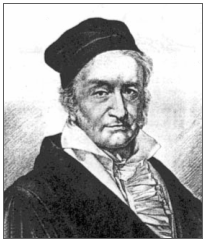This stream is ideal for students wanting to study mathematics according to the Ontario
academic mathematics curriculum. The material is covered at a level higher than that set by
most Ontario public and private schools, helping students transition to University-level
mathematics after high school. Each course can be completed in one semester.
Enriched Stream

This stream is ideal for students wanting to study mathematics at a challenging level according
to our curriculum. Students in this stream already excel in mathematics at school. Because of the
great depth of the material covered, each course takes one year to complete.
 Level
 Course Outline
 MAT-EP
 Number Theory, Whole Numbers, Factors and Multiples, Decimals and Fractions, Measurements, Integers, Operations, Algebra, Series and Sequences,Number Sense, Triangles, Quadrilaterals, Polygons, Circles
 MAT-E1
 Whole Numbers and Integers, Rational and Irrational Numbers, Exponents, Probabilities, Algebra, Equations, Inequations, Number Sense, Plane Geometry,3D Geometry, Perimeters, Rectilinear and Circular Areas, Volumes, Surface Areas
 MAT-E2
 Numeration, Problem Solving with Algebra, Equations with One and Two Variables, Number Sense with Algebra, Plane and 3D Geometry, Coordinates, Slopes,Equation of Lines, Parallel and Perpendicular Lines, Intersecting Lines
 MAT-E3
 Triangles and Trigonometry, Trigonometric Identities, The Sine and Cosine Laws,Linear Systems, Polynomials, Parabolas, Maximum and Minimum Values, Quadratic Inequations, Real and Complex Roots, Sigma Notation, Series and Sequences
 MAT-E4
 Inverse and Composite Functions, Polynomial Functions, Rational Functions,Absolute Value Functions, Trigonometric Identities and Equations, Exponential and Radical Functions, Logarithmic Functions, Circles and Conic Sections
 MAT-E5
 Vectors, Vector Arithmetic, Equations of Lines and Planes in 3D Space, Systems of Equations, Limits of Sequences, Infinite Series, Limits of Functions, Derivatives, Tangent Lines, Extreme Values, Curve Sketching, Indefinite and Definite Integrals,Areas and Volumes with Integrals

This stream is ideal for students wanting to study mathematics at a very high level according to
our curriculum. Students in this stream not only excel in mathematics at school, but typically
desire to pursue careers in engineering, computer science and physics. Because of the great
depth of the material covered, each course takes one year to complete.
 Level
 Course Outline
 MAT-GP
 Number Theory, Factors and Multiples, Decimals and Fractions, Probabilities, Integers, Algebra, Exponents, Rational and Irrational Numbers, Number Sense, Perimeters, Circumferences, Rectilinear and Circular Areas, Volumes and Surface Areas
 MAT-G1
 Real Numbers, Number Theory, Algebra, Equations and Inequations, Radicals,Number Sense, Plane Geometry, Pythagorean Theorem, Coordinates, Rectilinear and Circular Figures, 3D Geometry, Probabilities, Permutations and Combinations
 MAT-G2
 Factoring Trinomials, Equations and Inequations, Number Properties and Sets, Exponents and Radicals, Absolute Values, Analytic Geometry, Functions, Slopes, Equations of Lines, Quadratic Equations, Maximum and Minimum Values
 MAT-G3
 Linear Systems, Advanced Factoring, Divisibilities, Quadratic Inequations,Nature of Roots, Complex Numbers, Quadratic Systems, Equation of Circles,Tangents, Trigonometric Identities and Graphs, Discrete Functions, Sigma Notation
 MAT-G4
 Inverse and Composite Functions, Polynomial Functions, Rational Functions,Trigonometric Identities, Trigonometric Equations and Inequations, Exponential and Radical Functions, Logarithmic Functions, Conic Geometry and Equations
 MAT-G5
 Geometric and Algebraic Vectors, Equations of Lines and Planes in 3D Space, Limitsof Sequences, Infinite Series, Limits of Functions, Derivatives and their Applications,Curve Sketching, Indefinite and Definite Integrals, Areas and Volumes with Integrals